Next Article in Journal
Generalized (c,d)-Entropy and Aging Random Walks
Next Article in Special Issue
Entropy Diversity in Multi-Objective Particle Swarm Optimization
Previous Article in Journal
Competition of Dynamic Self-Confidence and Inhomogeneous Individual Influence in Voter Models
Previous Article in Special Issue
Diffusion in Relatively Homogeneous Sand Columns: A Scale-Dependent or Scale-Independent Process?
Open AccessArticle

# New Results on Fractional Power Series: Theories and Applications

byAhmad El-Ajou 1,Omar Abu Arqub 1,Zeyad Al Zhour 2 andShaher Momani 3,*1
Department of Mathematics, Faculty of Science, Al Balqa Applied University, Salt 19117, Jordan
2
Department of Basic Sciences and Humanities, College of Engineering, University of Dammam, Dammam 31451, KSA, Saudi Arabia
3
Department of Mathematics, Faculty of Science, University of Jordan, Amman 11942, Jordan
*
Author to whom correspondence should be addressed.
Entropy 2013, 15(12), 5305-5323; https://doi.org/10.3390/e15125305
Received: 12 September 2013 / Revised: 9 October 2013 / Accepted: 9 October 2013 / Published: 2 December 2013

## Abstract

In this paper, some theorems of the classical power series are generalized for the fractional power series. Some of these theorems are constructed by using Caputo fractional derivatives. Under some constraints, we proved that the Caputo fractional derivative can be expressed in terms of the ordinary derivative. A new construction of the generalized Taylor’s power series is obtained. Some applications including approximation of fractional derivatives and integrals of functions and solutions of linear and nonlinear fractional differential equations are also given. In the nonlinear case, the new and simple technique is used to find out the recurrence relation that determines the coefficients of the fractional power series.

## 1. Introduction

Fractional calculus theory is a mathematical analysis tool applied to the study of integrals and derivatives of arbitrary order, which unifies and generalizes the notions of integer-order differentiation and n-fold integration [1,2,3,4]. Commonly these fractional integrals and derivatives were not known to many scientists and up until recent years, they have been only used in a purely mathematical context, but during these last few decades these integrals and derivatives have been applied in many science contexts due to their frequent appearance in various applications in the fields of fluid mechanics, viscoelasticity, biology, physics, image processing, entropy theory, and engineering [5,6,7,8,9,10,11,12,13,14].
It is well known that the fractional order differential and integral operators are non-local operators. This is one reason why fractional calculus theory provides an excellent instrument for description of memory and hereditary properties of various physical processes. For example, half-order derivatives and integrals proved to be more useful for the formulation of certain electrochemical problems than the classical models [1,2,3,4]. Applying fractional calculus theory to entropy theory has also become a significant tool and a hotspot research domain [15,16,17,18,19,20,21,22,23,24] since the fractional entropy could be used in the formulation of algorithms for image segmentation where traditional Shannon entropy has presented limitations  and in the analysis of anomalous diffusion processes and fractional diffusion equations [19,20,21,22,23,24]. Therefore, the application of fractional calculus theory has become a focus of international academic research. Excellent accounts of the study of fractional calculus theory and its applications can be found in [25,26].
Power series have become a fundamental tool in the study of elementary functions and also other not so elementary ones as can be checked in any book of analysis. They have been widely used in computational science for easily obtaining an approximation of functions . In physics, chemistry, and many other sciences this power expansion has allowed scientist to make an approximate study of many systems, neglecting higher order terms around the equilibrium point. This is a fundamental tool to linearize a problem, which guarantees easy analysis [28,29,30,31,32,33,34,35].
The study of fractional derivatives presents great difficulty due to their complex integro-differential definition, which makes a simple manipulation with standard integer operators a complex operation that should be done carefully. The solution of fractional differential equations (FDEs), in most methods, appears as a series solution of fractional power series (FPS) [36,37,38,39,40,41,42]. Consequently, many authors suggest a general form of power series, specifically Taylor's series, including fractional ones. To mention a few, Riemann  has been written a formal version of the generalized Taylor series formula as:
$f ( x + h ) = ∑ m = − ∞ ∞ h m + r Γ ( m + r + 1 ) ( J s m + r f ) ( x ) ,$
where $J s m + r$ is the Riemann-Liouville fractional integral of order m + r. Watanabe in  has been obtained the following relation:
where $R n , k ( x ) = ( J s α + n D ^ s α + n f ) ( x ) + 1 Γ ( − α − k ) ∫ 0 x 0 ( x − t ) − α − k − 1 ( D ^ s α − k − 1 f ) ( t ) d t$ and $D ^ s α + n$ is the Riemann-Liouville fractional derivative of order α + n. Trujillo et al.  have been introduced the generalized Taylor's formula as:
where $R n ( x , x 0 ) = ( x − x 0 ) ( n + 1 ) α Γ ( ( n + 1 ) α + 1 ) ( D ^ s ( n + 1 ) α f ) ( ξ ) , x 0 ≤ ξ ≤ x$. Recently, Odibat and Shawagfeh  have been represented a new generalized Taylor's formula which as follows:
where and $D x 0 m α$ is the Caputo fractional derivative of order . For α = 1, the generalized Taylor's formula reduces to the classical Taylor's formula. Throughout this paper ℕ the set of natural numbers, ℝ the set of real numbers, and Γ is the Gamma function.
In this work, we dealt with FPS in general which is a generalization to the classical power series (CPS). Important theorems that related to the CPS have been generalized to the FPS. Some of these theorems are constructed by using Caputo fractional derivatives. These theorems have been used to approximate the fractional derivatives and integrals of functions. FPS solutions have been constructed for linear and nonlinear FDEs and a new technique is used to find out the coefficients of the FPS. Under certain conditions, we proved that the Caputo fractional derivative can be expressed in terms of the ordinary derivative. Also, the generalized Taylor's formula in Equation (4) has been derived using new approach for 0 ≤ m − 1 < αm , m ∈ ℕ.
The organization of this paper is as follows: in the next section, we present some necessary definitions and preliminary results that will be used in our work. In Section 3, theorems that represent the objective of the paper are mentioned and proved. In Section 4, some applications, including approximation of fractional derivatives and integrals of functions are given. In Section 5, series solutions of linear and nonlinear FDEs are produced using the FPS technique. The conclusions are given in the final part, Section 6.

## 2. Notations on Fractional Calculus Theory

In this section, we present some necessary definitions and essential results from fractional calculus theory. There are various definitions of fractional integration and differentiation, such as Grunwald-Letnikov's definition and Riemann-Liouville's definition [1,2,3,4]. The Riemann-Liouville derivative has certain disadvantages when trying to model real-world phenomena with FDEs. Therefore, we shall introduce a modified fractional differential operator $D s α$ proposed by Caputo in his work on the theory of viscoelasticity .
Definition 2.1: A real function f(x), x > 0 is said to be in the space Cμ, μ ∈ ℝ if there exists a real number ρ > μ such that f(x) = xρf1(x), where f1(x) ∈ C[0, ∞), and it is said to be in the space $C μ n$ if f(n) (x) ∈ Cμ, n ∈ ℕ.
Definition 2.2: The Riemann-Liouville fractional integral operator of order α ≥ 0 of a function f(x) ∈ Cμ, μ ≥ −1 is defined as:
Properties of the operator $J s α$ can be found in [1,2,3,4], we mention here only the following: for fCμ, μ ≥ −1, α, β ≥ 0, C ∈ ℝ, and γ ≥ −1, we have $J s α J s β f ( x ) = J s α + β f ( x ) = J s β J s α f ( x )$, $J s α C = C Γ ( α + 1 ) ( x − s ) α$, and $J s α ( x − s ) γ = Γ ( γ + 1 ) Γ ( α + γ + 1 ) ( x − s ) α + γ$.
Definition 2.3: The Riemann-Liouville fractional derivative of order α > 0 of is defined as:
$D ^ s α f ( x ) = { d n d x n J n − α f ( x ) , n − 1 < α < n , d n d x n f ( x ) , α = n .$
In the next definition we shall introduce a modified fractional differential operator $D s α$.
Definition 2.4: The Caputo fractional derivative of order α > 0 of is defined as:
$D s α f ( x ) = { J s n − α f ( n ) ( x ) , x > s ≥ 0 , n − 1 < α < n , d n f ( x ) d x n , α = n .$
For some certain properties of the operator $D s α$, it is obvious that when , and $C ∈ ℝ$, we have $D s α ( x − s ) γ = Γ ( γ + 1 ) Γ ( γ + 1 − α ) ( x − s ) γ − α$ and $D s α C = 0$.
Lemma 2.1: If $n − 1 < α ≤ n$, $f ∈ C μ n$, $n ∈ ℕ$, and $μ ≥ − 1$, then $D s α J s α f ( x ) = f ( x )$ and $J s α D s α f ( x ) = f ( x ) − ∑ j = 0 n − 1 f ( j ) ( s + ) ( x − s ) j j !$, where $x > s ≥ 0$.

## 3. Fractional Power Series Representation

In this section, we will generalize some important definitions and theorems related with the CPS into the fractional case in the sense of the Caputo definition. New results related to the convergent of the series $∑ n = 0 ∞ c n t n α$ are also presented. After that, some results which focus on the radii of convergence for the FPS are utilized.
The following definition is needed throughout this work, especially, in the following two sections regarding the approximating of the fractional derivatives, fractional integrals, and solution of FDEs.
Definition 3.1: A power series representation of the form
where 0 ≤ m − 1 < αm and tt0 is called a FPS about t0, where t is a variable and Cn’s are constants called the coefficients of the series.
As a special case, when t0 = 0 the expansion $∑ n = 0 ∞ c n t n α$ is called a fractional Maclaurin series. Notice that in writing out the term corresponding to n = 0 in Equation (8) we have adopted the convention that (tt0)0 = 1 even when t = t0. Also, when t = t0 each of the terms of Equation (8) vanishes for n ≥ 1 and so. On the other hand, the FPS (8) always converges when t = t0. For the sake of simplicity of our notation, we shall treat only the case where t0 = 0 in the first four theorems. This is not a loss of the generality, since the translation t’ = tt0 reduces the FPS about t0 to the FPS about 0.
Theorem 3.1: We have the following two cases for the FPS $∑ n = 0 ∞ c n t n α , t ≥ 0$:
(1)
If the FPS $∑ n = 0 ∞ c n t n α$ converges when $t = b > 0$, then it converges whenever $0 ≤ t < b$,
(2)
If the FPS $∑ n = 0 ∞ c n t n α$ diverges when $t = d > 0$, then it diverges whenever $t > d$.
Proof: For the first part, suppose that $∑ ​ c n b n α$ converges. Then, we have $lim n → ∞ c n b n α = 0$. According to the definition of limit of sequences with $ε = 1$, there is a positive integer $N$ such that $| c n b n α | < 1$ whenever $n ≥ N$. Thus, for $n ≥ N$, we have $| c n t n α | = | c n b n α t n α b n α | = | c n b n α | | t b | n α < | t b | n α$. Again, if $0 ≤ t < b$ , then $| t b | α < 1$, so $∑ ​ | t b | n α$ is a convergent geometric series. Therefore, by the comparison test, the series $∑ n = N ∞ | c n t n α |$ is convergent. Thus the series $∑ ​ c n t n α$ is absolutely convergent and therefore convergent. To prove the remaining part, suppose that $∑ ​ c n d n α$ diverges. Now, if $t$ is any number such that $t > d > 0$, then $∑ ​ c n t n α$ cannot converge because, by Case 1, the convergence of $∑ ​ c n t n α$ would imply the convergence of $∑ ​ c n d n α$. Therefore, $∑ ​ c n d n α$ diverges whenever $t > d$. This completes the proof.
Theorem 3.2: For the FPS $∑ n = 0 ∞ c n t n α , t ≥ 0$, there are only three possibilities:
(1)
The series converges only when $t = 0$,
(2)
The series converges for each $t ≥ 0$,
(3)
There is a positive real number such that the series converges whenever $0 ≤ t < R$ and diverges whenever $t > R$.
Proof: Suppose that neither Case 1 nor Case 2 is true. Then, there are nonzero numbers $b$ and $d$ such that $∑ ​ c n t n α$ converges for $t = b$ and diverges for $t = d .$ Therefore, the set $S = { t | ∑ ​ c n t n α$ converges } is not empty. By the preceding theorem, the series diverges if $t > d$, so $0 ≤ t ≤ d$ for each $t ∈ S$. This says that $d$ is an upper bound for $S$. Thus, by the completeness axiom, $S$ has a least upper bound $R$. If $t > R$, then $t ∉ S$, so $∑ ​ c n t n α$ diverges. If $0 ≤ t < R$, then $t$ is not an upper bound for $S$ and so there exists $b ∈ S$ such that $b > t$. Since $b ∈ S$ and $∑ ​ c n t n α$ converges, so by the preceding theorem $∑ ​ c n t n α$ converges, so the proof of the theorem is complete.
Remark 3.1: The number $R$ in Case 3 of Theorem 3.2 is called the radius of convergence of the FPS. By convention, the radius of convergence is in Case 1 and $R = ∞$ in Case 2.
Theorem 3.3: The CPS has radius of convergence if and only if the FPS has radius of convergence.
Proof: If we make the change of variable t = xα, x ≥ 0 then the CPS $∑ n = 0 ∞ c n t n$ becomes $∑ n = 0 ∞ c n x n α$. This series converges for 0 ≤ xα < R, that is for 0 ≤ x < R1/α, and so the FPS $∑ n = 0 ∞ c n x n α$ has radius of convergence R1/α. Conversely, if we make the change of variable t = x1/α, x ≥ 0 then the FPS $∑ n = 0 ∞ c n t n α$ becomes $∑ n = 0 ∞ c n x n$. In fact, this series converges for 0 ≤ x1/α < R1/α that is for 0 ≤ x < R. Since the two series and $∑ n = 0 ∞ c n x n ,$ −∞ < x < ∞ have the same radius of convergence $R = lim n → ∞ | c n c n + 1 |$, the radius of convergence for the CPS $∑ n = 0 ∞ c n x n ,$ −∞ < x < ∞ is R, so the proof of the theorem is complete.
Theorem 3.4: Suppose that the FPS $∑ n = 0 ∞ c n t n α$ has radius of convergence. If $f ( t )$ is a function defined by $f ( t ) = ∑ n = 0 ∞ c n t n α$ on 0 ≤ t < R, then for 0 ≤ m − 1 < α < m and 0 ≤ t < R, we have:
$D 0 α f ( t ) = ∑ n = 1 ∞ c n Γ ( n α + 1 ) Γ ( ( n − 1 ) α + 1 ) t ( n − 1 ) α ,$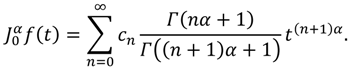Proof: Define $g ( x ) = ∑ n = 0 ∞ c n x n$ for 0 ≤ x < Rα, where Rα is the radius of convergence. Then:where 0 ≤ τ < x < Rα. On the other hand, if we make the change of variable x = tα, t ≥ 0 into Equation (11) and use the properties of the operator $D 0 α$, we obtain:For the remaining part, considering the definition of g(x) above one can conclude that:where 0 ≤ τ < x < Rα. Similarly, if we make the change of variable x = tα, t ≥ 0 into Equation (13), we can conclude that: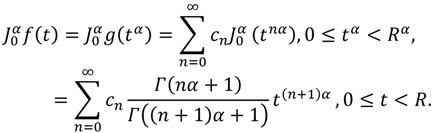So the proof of the theorem is complete.
Theorem 3.5: Suppose that f has a FPS representation at t0 of the form:If f(t) ∈ C [t0, t0 + R) and for n = 0,1,2,…, then the coefficients cn in Equation (15) will take the form $c n = D t 0 n α f ( t 0 ) Γ ( n α + 1 )$, where $D t 0 n α = D t 0 α · D t 0 α · … · D t 0 α$ (n-times).
Proof: Assume that f is an arbitrary function that can be represented by a FPS expansion. First of all, notice that if we put t = t0 into Equation (15), then each term after the first vanishes and thus we get c0 = f(t0). On the other aspect as well, by using Equation (9), we have:
$D t 0 α f ( t ) = c 1 Γ ( α + 1 ) + c 2 Γ ( 2 α + 1 ) Γ ( α + 1 ) ( t − t 0 ) α + c 3 Γ ( 3 α + 1 ) Γ ( 2 α + 1 ) ( t − t 0 ) 2 α + … ,$
where t0t < t0 + R. The substitution of t = t0 into Equation (16) leads to $c 1 = D t 0 α f ( t 0 ) Γ ( α + 1 )$. Again, by applying Equation (9) on the series representation in Equation (16), one can obtain that:
$D 0 2 α f ( t ) = c 2 Γ ( 2 α + 1 ) + c 3 Γ ( 3 α + 1 ) Γ ( α + 1 ) ( t − t 0 ) α + c 4 Γ ( 4 α + 1 ) Γ ( 2 α + 1 ) ( t − t 0 ) 2 α + … ,$
where t0t < t0 + R. Here, if we put t = t0 into Equation (17), then the obtained result will be $c 2 = D t 0 2 α f ( t 0 ) Γ ( 2 α + 1 )$. By now we can see the pattern and discover the general formula for cn. However, if we continue to operate $D t 0 α$(∙) n-times and substitute t = t0, we can get . This completes the proof.
We mention here that the substituting of back into the series representation of Equation (15) will leads to the following expansion for f about t0:
which is the same of the Generalized Taylor's series that obtained in  for 0 < α ≤ 1.
Theorem 3.6: Suppose that f has a Generalized Taylor's series representation at t0 of the form:
If $D t 0 n α f ( t ) ∈ C ( t 0 , t 0 + R )$ for $n = 0 , 1 , 2 , … ,$ then $D t 0 n α f ( t 0 ) = Γ ( n α + 1 ) n ! g ( n ) ( t 0 ) ,$ where $g ( t ) = f ( ( t − t 0 ) 1 / α + t 0 )$, $t 0 ≤ t < t 0 + R α$.
Proof: If we make the change of variable $t = ( x − t 0 ) 1 / α + t 0$, $t 0 ≤ x < t 0 + R α$ into Equation (19), then we obtain:
But since, the CPS representation of g(x) about t0 takes the form:
Then the two power series expansion in Equations (20) and (21) converge to the same function g(x). Therefore, the corresponding coefficients must be equal and thus $D t 0 n α f ( t 0 ) = Γ ( n α + 1 ) n ! g ( n ) ( t 0 )$. This completes the proof.
As with any convergent series, this means that f(t) is the limit of the sequence of partial sums. In the case of the Generalized Taylor's series, the partial sums are $T n ( t ) = ∑ j = 0 n D t 0 j α f ( t 0 ) Γ ( j α + 1 ) ( t − t 0 ) j α$. In general, f(t) is the sum of its Generalized Taylor's series if $f ( t ) = lim n → ∞ T n ( t )$. On the other aspect as well, if we let Rn(t) = f(t) − Tn(t), then Rn(t) is the remainder of the Generalized Taylor's series.
Theorem 3.7: Suppose that f(t) ∈ C[t0, t0 + R) and $D t 0 j α f ( t ) ∈ C ( t 0 , t 0 + R )$ for j = 0,1,2,…, n+ 1, where 0 < α ≤ 1. Then f could be represented by:
$f ( t ) = ∑ j = 0 n ( D t 0 j α f ) ( t 0 ) Γ ( j α + 1 ) ( t − t 0 ) j α + J t 0 ( n + 1 ) α D t 0 ( n + 1 ) α f ( t ) , t 0 ≤ t ≤ t 0 + R .$
Proof: From the certain properties of the operator $J α α$ and Lemma 2.1, one can find that:If we keep repeating of this process, then after n-times of computations, we can find that $J t 0 ( n + 1 ) α D t 0 ( n + 1 ) α f ( t ) = f ( t ) − ∑ j = 0 n ( D t 0 j α f ) ( t 0 ) Γ ( j α + 1 ) ( t − t 0 ) j α , t 0 ≤ t ≤ t 0 + R$, so the proof of the theorem is complete.
Theorem 3.8: If $| D t 0 ( n + 1 ) α f ( t ) | ≤ M$ on t0td, where 0 < α ≤ 1, then the reminder Rn(t) of the Generalized Taylor's series will satisfies the inequality:
$| R n ( t ) | ≤ M Γ ( ( n + 1 ) α + 1 ) ( t − t 0 ) ( n + 1 ) α , t 0 ≤ t ≤ d .$
Proof: First of all, assume that $D t 0 j α f ( t )$ exist for $j = 0 , 1 , 2 , … , n + 1$ and that:
$| D t 0 ( n + 1 ) α f ( t ) | ≤ M , t 0 ≤ t ≤ d .$
From the definition of the reminder $R n ( t ) = f ( t ) − ∑ j = 0 n D t 0 j α f ( t 0 ) Γ ( j α + 1 ) ( t − t 0 ) j α$ one can obtain $R n ( t 0 ) = D t 0 α R n ( t 0 ) = D t 0 2 α R n ( t 0 ) = … = D t 0 n α R n ( t 0 ) = 0$ and $D t 0 ( n + 1 ) α R n ( t ) = D t 0 ( n + 1 ) α f ( t ) , t 0 ≤ t ≤ d .$ It follows from Equation (25) that $| D t 0 ( n + 1 ) α f ( t ) | ≤ M$. Hence, $− M ≤ D t 0 ( n + 1 ) α f ( t ) ≤ M , t 0 ≤ t ≤ d$. On the other hand, we have:But since from Theorem 3.7, we get $J t 0 ( n + 1 ) α D t 0 ( n + 1 ) α f ( t ) = R n ( t )$. Thus, by performing the operations in Equation (26), we can find the inequality $− M ( t − t 0 ) ( n + 1 ) α Γ ( ( n + 1 ) α + 1 ) ≤ R n ( t ) ≤ M ( t − t 0 ) ( n + 1 ) α Γ ( ( n + 1 ) α + 1 ) , t 0 ≤ t ≤ d$ which is equivalent to $| R n ( t ) | ≤ M Γ ( ( n + 1 ) α + 1 ) ( t − t 0 ) ( n + 1 ) α , t 0 ≤ t ≤ d$, so the proof of the theorem is complete.
Theorem 3.9: Suppose that $f$ has a FPS representation at $t 0$ of the form
where R is the radius of convergence. Then f is analytic in (t0, t0 + R)
Proof: Let and h(t) = (tt0)α, t0t < t0 + R, 0 ≤ m − 1 < αm. Then g(t) and h(t) are analytic functions and thus the composition (gh)(t) = f(t) is analytic in (t0, t0 + R). This completes the proof.

## 4. Application I: Approximation Fractional Derivatives and Integrals of Functions

In order to illustrate the performance of the presented results in approximating the fractional derivatives and integrals of functions at a given point we consider two examples. On the other hand, we use Theorems 3.4, 3.6, and the generalized Taylor's series (18) in the approximation step. However, results obtained are found to be in good agreement with each other. In the computation process all the symbolic and numerical computations were performed using the Mathematica 7 software packages.
Application 4.1: Consider the following non-elementary function:
The fractional Maclaurin series representation of f(t) about t = 0 is . According to Theorem 3.6, we can conclude that , where $g ( t ) = f ( t 1 / α ) = 1 1 − t$ and g(n)(0) = n!. In other words, the fractional Maclaurin series of $f ( t )$ can be written as . In fact, this is a convergent geometric series with ratio $t α$. Thus, the series is convergent for each $0 ≤ t α < 1$ and then for each $0 ≤ t < 1$. Therefore is the sum of its fractional Maclaurin series representation. Note that, this result can be used to approximate the functions $D 0 α f ( t )$ and $J 0 α f ( t )$ on $0 ≤ t < 1$ However, according to Equation (9), the function $D 0 α f ( t )$ can be approximated by the $k th$-partial sum of its expansion as follows:
Our next goal is to approximate the function $D 0 α f ( t )$ in numerical values. To do so, Table 1 shows approximate values of $D 0 α f ( t )$ for different values of t and α on $0 ≤ t < 1$ in step of 0.1 when $k = 10$. It is to be noted that in order to improve the results, we can compute more approximation terms for different values of $t$ and $α$.
Similarly, we can use Equation (10) to approximate the function $J 0 α f ( t )$ in numerical values by the $k th$-partial sum of its expansion as:
Table 2 shows approximate values of $D 0 α f ( t )$ for different values of t and α on 0 ≤ t < 1 in step of 0.1 when k = 10. As in the previous table and results, it should to be noted that computing more terms of the series representation will increase the accuracy of the approximations and thus a good approximation can be obtained.
Table 1. The approximate values of $D 0 α f ( t )$ when k = 10 for Application 4.1.
Table 1. The approximate values of $D 0 α f ( t )$ when k = 10 for Application 4.1.
t α = 0.5 α = 0.75 α = 1.5 α = 2
0 0.886227 0.919063 1.329340 2
0.1 1.448770 1.253617 1.481250 2.123057
0.2 1.918073 1.619507 1.814089 2.531829
0.3 2.499525 2.113559 2.385670 3.370618
0.4 3.261329 2.825448 3.371164 4.994055
0.5 4.277607 3.899429 5.179401 8.295670
0.6 5.635511 5.569142 8.843582 15.839711
0.7 9.803122 8.201646 17.203979 36.370913
0.8 9.803122 12.353432 38.389328 104.441813
0.9 12.869091 18.839971 95.486744 365.976156
Table 2. The approximate values of $J 0 α f ( t )$ when k = 10 for Application 4.1.
Table 2. The approximate values of $J 0 α f ( t )$ when k = 10 for Application 4.1.
t α = 0.5 α = 0.75 α = 1.5 α = 2
0 0 0 0 0
0.1 0.121746 0.025296 0.025296 0.000008
0.2 0.289460 0.080361 0.001859 0.000136
0.3 0.509120 0.165975 0.006551 0.000700
0.4 0.795368 0.289398 0.016399 0.002283
0.5 1.169853 0.463570 0.034285 0.005812
0.6 1.662260 0.709964 0.064487 0.012745
0.7 2.311743 1.063626 0.114028 0.025437
0.8 3.168540 1.580987 0.195965 0.048045
0.9 4.295695 2.351284 0.338186 0.089143
Application 4.2: Consider the following Mittag-Leffler function: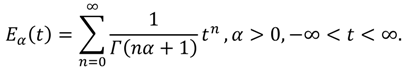The Mittag-Leffler function  plays a very important role in the solution of linear FDEs [3,8]. In fact, the solutions of such FDEs are obtained in terms of Eα(tα). Note that $D 0 n α$(Eα(tα)) ∈ C(0, ∞) for n ∈ ℕ and α > 0. In  the authors have approximated the function Eα(tα) for different values of t when 0 < α ≤ 1 by 10-th partial sum of its expansion. However, using Equations (9) and (10) both functions $D 0 α$(Eα(tα)) and $J 0 α$(Eα(tα)) can be approximated, respectively, by the following k-partial sums:Again, to show the validity of our FPS representation in approximating the Mittag-Leffler function, Table 3 and Table 4 will tabulate the approximate results of $D 0 α$(Eα(tα)) and J(Eα(tα)) for different values of t and α on 0 ≤ t ≤ 4 in step of 0.4 when k = 10.
Table 3. The approximate values of $D 0 α$(Eα(tα)) when k = 10 for Application 4.2.
Table 3. The approximate values of $D 0 α$(Eα(tα)) when k = 10 for Application 4.2.
t α = 0.5 α = 0.75 α = 1.5 α = 2
0 1 1 1 1
0.4 2.430013 1.800456 1.201288 1.081072
0.8 3.991267 2.816662 1.630979 1.337435
1.2 6.220864 4.298057 2.324700 1.810656
1.6 9.451036 6.489464 3.389416 2.577464
2.0 14.097234 9.743204 4.996647 3.762196
2.4 20.683136 14.543009 7.407121 5.556947
2.8 29.857007 21.721976 11.012177 8.252728
3.2 42.406132 32.250660 16.396938 12.286646
3.6 59.270535 47.649543 24.435073 18.312779
4.0 81.556340 69.980001 36.430382 27.308232
Table 4. The approximate values of $J 0 α$(Eα(tα))when k = 10 for Application 4.2.
Table 4. The approximate values of $J 0 α$(Eα(tα))when k = 10 for Application 4.2.
t α = 0.5 α = 0.75 α = 1.5 α = 2
0 0 0 0 0
0.4 1.430036 0.800456 0.201288 0.081072
0.8 2.992286 1.816664 0.630979 0.337435
1.2 5.230333 3.298122 1.324701 0.810656
1.6 8.497108 5.490163 2.389416 1.577464
2.0 13.254431 8.747610 3.996647 2.762196
2.4 20.111627 13.592834 6.407121 4.556947
2.8 29.857352 20.792695 10.012177 7.252728
3.2 43.491129 31.463460 15.396941 11.286646
3.6 62.255682 47.211856 23.435091 17.312779
4.0 87.670285 70.321152 35.430382 26.308232

## 5. Application II: Series Solutions of Fractional Differential Equations

In this section, we use the FPS technique to solve the FDEs subject to given initial conditions. This method is not new, but it is a powerful application on the theorems in this work. Moreover, a new technique is applied on the nonlinear FDEs to find out the recurrence relation which gives the value of coefficients of the FPS solution as we will see in Applications (5.3) and (5.4).
Application 5.1: Consider the following linear fractional equation :subject to the initial conditions: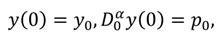where ω, y0, and ρ0 are real finite constants.
The FPS technique consists in expressing the solution of Equations (33) and (34) as a FPS expansion about the initial point t = 0. To achieve our goal, we suppose that this solution takes the form of Equation (8) which is:From formula (9), we can obtain. On the other hand, it easy to see that:In order to approximate the solution of Equations (33) and (34) substitute the expansion formulas of Equations (35) and (36) into Equation (33), yields that:The equating of the coefficients of t to zero in both sides of Equation (37) leads to the following:. Considering the initial conditions (34) one can obtain c0 = y0 and. In fact, based on these results the remaining coefficients of t can be divided into two categories. The even index terms and the odd index terms, where the even index terms take the formand so on, and the odd index term which are, and so on. Therefore, we can obtain the following series expansion solution:On the other aspect as well, the exact solution of Equations (33) and (34) in term of the Mittag-Leffler function has the general form which are coinciding with the exact solution:Application 5.2: Consider the following composite linear fractional equation :subject to the initial conditions:Using FPS technique and considering formula (8), the solution y(t) of Equations (40) and (41) can be written as:In order to complete the formulation of the FPS technique, we must compute the functions. However, the forms of these functions are giving, respectively, as follows:But since {t|t ≥ 0} is the domain of solution, then the values of the coefficients c1 and c3 must be zeros. On the other aspect as well, the substituting of the initial conditions (41) into Equation (42) and into $D 0 1$y(t) in Equation (43) gives c0 = 0 and c2 = 0. Therefore, the discretized form of the functions y(t), $D 0 1/2$y(t), and $D 0 2$y(t) is obtained. The resulting new form will be as follows: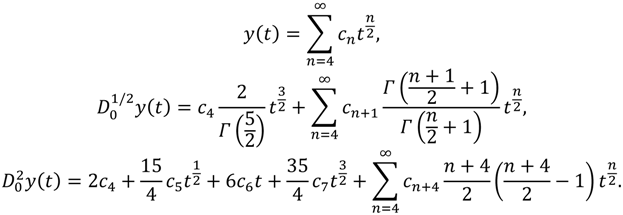Now, substituting Equation (44) back into Equation (40), equating the coefficients of tn/2 to zero in the resulting equation, and finally identifying the coefficients, we then will obtain recursively the following results:, and. So, the 15th-truncated series approximation of y(t) is:The FPS technique has an advantage that it is possible to pick any point in the interval of integration and as well the approximate solution and all its derivatives will be applicable. In other words a continuous approximate solution will be obtained. Anyway, Tables 5 shows the 15th-approximate values of y(t), $D 0 1/2$y(t), $D 0 2$y(t) and and the residual error function for different values of t on 0 ≤ t ≤ 1 in step of 0.2, where the residual error function is defined as.
Table 5. The 15th-approximate values of y(t), $D 0 1/2$y(t), and $D 0 2$y(t) and Res(t) for Application 5.2.
Table 5. The 15th-approximate values of y(t), $D 0 1/2$y(t), and $D 0 2$y(t) and Res(t) for Application 5.2.
t y(t) $D 0 1/2$y(t) $D 0 2$y(t) Res(t)
0.0 0 0 0 0
0.2 0.157037 0.525296 7.317668 6.211481 × 10−7
0.4 0.604695 1.413213 5.982030 6.167617 × 10−5
0.6 1.290452 2.420120 4.288506 9.217035 × 10−4
0.8 2.1472288 3.409426 2.437018 6.327666 × 10−3
1.0 3.101501 4.282177 0.587987 2.833472 × 10−2
From the table above, it can be seen that the FPS technique provides us with the accurate approximate solution for Equations (40) and (41). Also, we can note that the approximate solution more accurate at the beginning values of the independent interval.
Application 5.3: Consider the following nonlinear fractional equation :subject to the initial conditions:where m is a positive integer number.
Similar to the previous discussions, the FPS solution takes the form. On the other hand, according to the initial conditions (47), the coefficient c0 must be equal to zero. Therefore: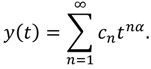It is known that in the nonlinear FDEs case the finding of recurrence relation that corresponding to the FPS representation and then discovering the values of the coefficients is not easy in general. Therefore, a new technique will be used in this application in order to find out the value of the coefficients of the FPS solution. To achieve our goal, we define the so-called αkth-order differential equation as follows: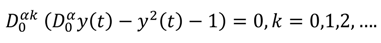It is obvious that when k = 0, Equation (49) is the same as Equation (46). So, the FPS representation in Equation (48) is a solution for the αkth-order differential Equation (49); that is:According to Equation (9) a new discretized version of Equation (50) will be obtained and is given as:where Xk = 1 if k = 0 and Xk = 0 if k ≥ 1. From Theorems 3.2 and 3.4, the αkth-derivative of the FPS representation, Equation (48), is convergent at least at t = 0, for k = 0,1,2,…. Therefore, the substituting t = 0 into Equation (51) gives the following recurrence relation which determine the values of the coefficients cn of t: c0 = 0, $c 1 = 1 r ( α + 1 )$, and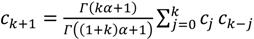for k = ,1,2,…. If we collect and substitute these value of the coefficients back into Equation (48), then the exact solution of Equations (46) and (47) has the general form which is coinciding with the general expansion:In fact, these coefficients are the same as coefficients of the series solution that obtained by the Adomian decomposition method . Moreover, if α = 1, then the series solution for Equations (46) and (47) will be:which agrees well with the exact solution of Equations (46) and (47) in the ordinary sense.
Table 6 shows the 15th-approximate values of y(t) and the residual error function for different values of t and α on 0 ≤ t ≤ 1 in step of 0.2, where the residual error function is defined as. However, the computational results below provide a numerical estimate for the convergence of the FPS technique. It is also clear that the accuracy obtained using the present technique is advanced by using only a few approximation terms. In addition, we can conclude that higher accuracy can be achieved by evaluating more components of the solution. In fact, the results reported in this table confirm the effectiveness and good accuracy of the technique.
Application 5.4: Consider the following composite nonlinear fractional equation :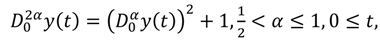subject to the initial conditions:where c0 and c1 are real finite constants.
Table 6. The 15th-approximate values of y(t) and Res(t) for Application 5.3.
Table 6. The 15th-approximate values of y(t) and Res(t) for Application 5.3.
t y(t;α = 1.5) Res(t;α = 1.5) y(t;α = 2.5) Res(t;α = 2.5)
0.0 0 0 0 0
0.2 0.067330 2.034437 × 10−17 0.005383 3.103055 × 10−16
0.4 0.191362 4.370361 × 10−17 0.030450 1.252591 × 10−15
0.6 0.356238 2.850815 × 10−13 0.083925 7.275543 × 10−16
0.8 0.563007 2.897717 × 10−10 0.172391 1.022700 × 10−15
1.0 0.822511 6.341391 × 10−8 0.301676 1.998026 × 10−16
Again, using FPS expansion, we assume that the solution y(t). of Equations (54) and (55) can be expanded in the form of $y ( t ) = ∑ n = 0 ∞ c n t n α$. Thus, the so-called $α k$th-order differential equation of Equations (54) and (55) is:
According to Equation (9) and the Cauchy product for infinite series, the discretized form of Equation (56) is obtained as follows:In fact, Equation (57) can be easily reduces depending on Equation (9) once more into the equivalent form as:where $χ k = 1$ if $k = 0$ and $χ k = 0$ if $k ≥ 1$. However, the substituting of $t = 0$ into Equation (58) gives the following recurrence relation which determines the values of the coefficients $c n$ of $t n α$: c0 and c1 are arbitrary, $c 2 = Γ ( 2 α + 1 ) 1 + c 1 2 ( Γ ( α + 1 ) ) 2$ and $c k + 2 = Γ ( k α + 1 ) Γ ( ( 2 + k ) α + 1 ) ∑ j = 0 k c j + 1 c k − j + 1 Γ ( ( j + 1 ) α + 1 ) Γ ( j α + 1 ) Γ ( ( k − j + 1 ) α + 1 ) Γ ( ( k − j ) α + 1 )$ for $k = 1 , 2 , …$. Therefore, by easy calculations we can obtain that the general solution of Equations (54) and (55) agree well with the following expansion: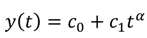For easy calculations and new generalization, one can assigns some specific values for the two constant c0 and c1 in the set of real or complex numbers.

## 6. Conclusions

The fundamental goal of this work has been to generalize the main theorems of the CPS into the FPS. The goal has been achieved successfully, whereby the Caputo fractional derivatives definition has been used to construct some of these theorems and relations. A Generalized Taylor's formula derived by some authors for 0 < α ≤ 1 can now be circulated for m − 1 < αm, m ∈ ℕ. Fractional derivatives are written in terms of ordinary derivatives under some constraints and we hope that in the future, this result can be achieved without any constraints. The theorems which have been proved in this paper are used to approximate the fractional derivatives and integrals of functions that can be written as a FPS representation. These theorems may simplify and modify some of the methods used to solve FDEs and fractional integro-differential equations such as differential transform method, homotopy analysis method, Adomian decomposition method, and others.

## Acknowledgments

The authors would like to express their thanks to unknown referees for their careful reading and helpful comments.

## Conflicts of Interest

The authors declare no conflict of interest.

## References

1. Mainardi, F. Fractional calculus: Some basic problems in continuum and statistical mechanics. In Fractals and Fractional Calculus in Continuum Mechanics; Carpinteri, A., Mainardi, F., Eds.; Springer-Verlag: Wien, Austria, 1997; pp. 291–348. [Google Scholar]
2. Miller, K.S.; Ross, B. An Introduction to the Fractional Calculus and Fractional Differential Equations; John Willy and Sons, Inc.: New York, NY, USA, 1993. [Google Scholar]
3. Oldham, K.B.; Spanier, J. The Fractional Calculus; Academic Press: New York, NY, USA, 1974. [Google Scholar]
4. Podlubny, I. Fractional Differential Equations; Academic Press: New York, NY, USA, 1999. [Google Scholar]
5. Beyer, H.; Kempfle, S. Definition of physical consistent damping laws with fractional derivatives. Z. Angew. Math. Mech. 1995, 75, 623–635. [Google Scholar] [CrossRef]
6. He, J.H. Some applications of nonlinear fractional differential equations and their approximations. Sci. Technol. Soc. 1999, 15, 86–90. [Google Scholar]
7. He, J.H. Approximate analytic solution for seepage flow with fractional derivatives in porous media. Comput. Method. Appl. M. 1998, 167, 57–68. [Google Scholar] [CrossRef]
8. Caputo, M. Linear models of dissipation whose Q is almost frequency independent-II. Geophys. J. Int. 1967, 13, 529–539. [Google Scholar] [CrossRef]
9. Yan, J.P.; Li, C.P. On chaos synchronization of fractional differential equations. Chaos, Solitons Fractals 2007, 32, 725–735. [Google Scholar] [CrossRef]
10. Sommacal, L.; Melchior, P.; Dossat, A.; Petit, J.; Cabelguen, J.M.; Oustaloup, A.; Ijspeert, A.J. Improvement of the muscle fractional multimodel for low-rate stimulation. Biomed. Signal Process. Control 2007, 2, 226–233. [Google Scholar] [CrossRef]
11. Silva, M.F.; Machado, J.A.T.; Lopes, A.M. Fractional order control of a hexapod robot. Nonlinear Dyn. 2004, 38, 417–433. [Google Scholar] [CrossRef]
12. Mathieu, B.; Melchior, P.; Oustaloup, A.; Ceyral, C. Fractional differentiation for edge detection. Signal Process. 2003, 83, 2421–2432. [Google Scholar] [CrossRef]
13. Zunino, L.; Pérez, D.G.; Martín, M.T.; Garavaglia, M.; Plastino, A.; Rosso, O.A. Permutation entropy of fractional Brownian motion and fractional Gaussian noise. Phys. Lett. A 2008, 372, 4768–4774. [Google Scholar] [CrossRef]
14. Jumarie, G. Path probability of random fractional systems defined by white noises in coarse-grained time applications of fractional entropy. Frac. Diff. Eq. 2011, 1, 45–87. [Google Scholar] [CrossRef]
15. Ubriaco, M.R. Entropies based on fractional calculus. Phys. Lett. A 2009, 373, 2516–2519. [Google Scholar] [CrossRef]
16. Li, H.; Haldane, F.D.M. Entanglement spectrum as a generalization of entanglement entropy: Identification of topological order in non-abelian fractional quantum hall effect states. Phys. Rev. Lett. 2008, 101, 010504. [Google Scholar] [CrossRef] [PubMed]
17. Hoffmann, K.H.; Essex, C.; Schulzky, C. Fractional diffusion and entropy production. J. Non-Equilib. Thermodyn. 1998, 23, 166–175. [Google Scholar] [CrossRef]
18. Machado, J.A.T. Entropy analysis of integer and fractional dynamical systems. Nonlinear Dyn. 2010, 62, 371–378. [Google Scholar]
19. Essex, C.; Schulzky, C.; Franz, A.; Hoffmann, K.H. Tsallis and Rényi entropies in fractional diffusion and entropy production. Physica A 2000, 284, 299–308. [Google Scholar] [CrossRef]
20. Cifani, S.; Jakobsen, E.R. Entropy solution theory for fractional degenerate convection–diffusion equations. Ann. Inst. Henri Poincare C, Non Linear Anal. 2011, 28, 413–441. [Google Scholar] [CrossRef][Green Version]
21. Prehl, J.; Essex, C.; Hoffmann, K.H. Tsallis relative entropy and anomalous diffusion. Entropy 2012, 14, 701–706. [Google Scholar] [CrossRef]
22. Prehl, J.; Boldt, F.; Essex, C.; Hoffmann, K.H. Time evolution of relative entropies for anomalous diffusion. Entropy 2013, 15, 2989–3006. [Google Scholar] [CrossRef]
23. Prehl, J.; Essex, C.; Hoffmann, K.H. The superdiffusion entropy production paradox in the space-fractional case for extended entropies. Physica A 2010, 389, 215–224. [Google Scholar] [CrossRef]
24. Magin, R.L.; Ingo, C.; Colon-Perez, L.; Triplett, W.; Mareci, T.H. Characterization of anomalous diffusion in porous biological tissues using fractional order derivatives and entropy. Microporous Mesoporous Mater. 2013, 178, 39–43. [Google Scholar] [CrossRef] [PubMed]
25. Kilbas, A.A.; Srivastava, H.M.; Trujillo, J.J. Theory and Applications of Fractional Differential Equations; vol. 204 of North-Holland Mathematics Studies; Elsevier Science B.V.: Amsterdam, The Netherlands, 2006. [Google Scholar]
26. Lakshmikantham, V.; Leela, S.; Vasundhara Devi, J. Theory of Fractional Dynamic Systems; Cambridge Academic: Cambridge, UK, 2009. [Google Scholar]
27. Apostol, T. Calculus; Blaisdell Publishing: Waltham, MA, USA, 1990. [Google Scholar]
28. Chang, Y.F.; Corliss, G. ATOMFT: Solving ODE's and DAE's using Taylor series. Comput. Math. Appl. 1994, 28, 209–233. [Google Scholar] [CrossRef]
29. Sezer, M.; Daşcioğlu, A.A. A Taylor method for numerical solution of generalized pantograph equations with linear functional argument. J. Comput. Appl. Math. 2007, 200, 217–225. [Google Scholar] [CrossRef]
30. Yalҫinbaş, S.; Sezer, M. The approximate solution of high-order linear Volterra-Fredholm integro-differential equations in terms of Taylor polynomials. Appl. Math. Comput. 2000, 112, 291–308. [Google Scholar] [CrossRef]
31. Abu Arqub, O.; Abo-Hammour, Z.; Al-badarneh, R.; Momani, S. A reliable analytical method for solving higher-order initial value problems. Discrete Dyn. Nat. Soc. 2014. [Google Scholar] [CrossRef]
32. Abu Arqub, O.; El-Ajou, A.; Bataineh, A.; Hashim, I. A representation of the exact solution of generalized Lane-Emden equations using a new analytical method. Abstr. Appl. Anal. 2013. [Google Scholar] [CrossRef]
33. Abu Arqub, O. Series solution of fuzzy differential equations under strongly generalized differentiability. J. Adv. Res. Appl. Math. 2013, 5, 31–52. [Google Scholar] [CrossRef]
34. El-Ajou, A.; Abu Arqub, O.; Momani, S. Homotopy analysis method for second-order boundary value problems of integrodifferential equations. Discrete Dyn. Nat. Soc. 2012. [Google Scholar] [CrossRef]
35. Abu Arqub, O.; El-Ajou, A.; Momani, S.; Shawagfeh, N. Analytical solutions of fuzzy initial value problems by HAM. Appl. Math. Inf. Sci. 2013, 7, 1903–1919. [Google Scholar] [CrossRef]
36. El-Ajou, A.; Odibat, Z.; Momani, S.; Alawneh, A. Construction of analytical solutions to fractional differential equations using homotopy analysis method. Int. J. Appl. Math. 2010, 40, 43–51. [Google Scholar]
37. El-Ajou, A.; Abu Arqub, O.; Momani, S. Solving fractional two-point boundary value problems using continuous analytic method. Ain Shams Eng. J. 2013, 4, 539–547. [Google Scholar] [CrossRef]
38. Abu Arqub, O.; El-Ajou, A. Solution of the fractional epidemic model by homotopy analysis method. J. King Saud Univ. Sci. 2013, 25, 73–81. [Google Scholar] [CrossRef]
39. Momani, S.; Odibat, Z. Numerical comparison of methods for solving linear differential equations of fractional order. Chaos, Solitons Fractals 2007, 31, 1248–1255. [Google Scholar] [CrossRef]
40. Shawagfeh, N. Analytical approximate solutions for nonlinear fractional differential equations. Appl. Math. Comput. 2002, 131, 517–529. [Google Scholar] [CrossRef]
41. Odibat, Z.; Momani, S. Application of variation iteration method to nonlinear differential equations of fractional order. Int. J. Nonlinear Sci. Numer. Simul. 2006, 7, 27–34. [Google Scholar] [CrossRef]
42. He, J.H. Homotopy perturbation method for bifurcation of nonlinear problems. Int. J. Nonlinear Sci. Numer. Simul. 2005, 6, 207–208. [Google Scholar] [CrossRef]
43. Hardy, G. Riemann's form of Taylor series. J. London Math. Soc. 1945, 20, 48–57. [Google Scholar] [CrossRef]
44. Wantanable, Y. Notes on the generalized derivatives of Riemann-Liouville and its application to Leibntz's formula. Tohoku Math. J. 1931, 24, 8–41. [Google Scholar]
45. Truilljo, J.; Rivero, M.; Bonilla, B. On a Riemann-Liouville generalized Taylor's formula. J. Math. Anal. Appl. 1999, 231, 255–265. [Google Scholar]
46. Odibat, Z.; Shawagfeh, N. Generalized Taylor’s formula. Appl. Math. Comput. 2007, 186, 286–293. [Google Scholar] [CrossRef]
47. Schneider, W.R. Completely monotone generalized Mittag-Leffler functions. Expo. Math. 1996, 14, 3–16. [Google Scholar]
48. Turchetti, G.; Usero, D.; Vazquez, L. Fractional derivative and Hamiltonian systems. Tamsui Oxford J. Math. Sci. 2002, 18, 45–56. [Google Scholar]